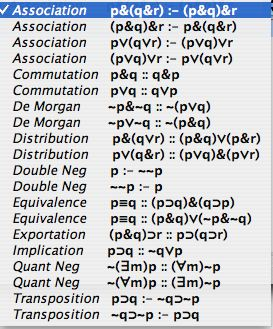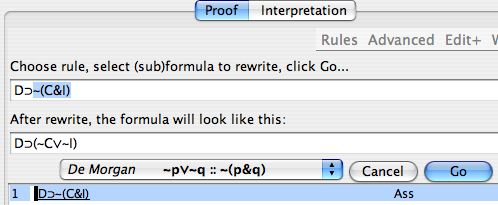# Tutorial 10: Common Inference Patterns and Rewrite Rules

Logical System

2013

### Skills to be acquired

Becoming familiar with common inference patterns and being able to use them via three new rules of inference and via rewrite rules. This helps with assessing ordinary everyday reasoning such as that found in the law, in newspapers, in advertisements, etc.

Bergmann The Logic Book Section 5.5

### The Tutorial:

First, a little background... Introductory logic is often taught using one of two different styles of sets of inference rules. There is what we have done here, using 'Introlim' rules, where every connective has an introduction rule and an elimination rule, and all rules work on the main connective of a formula. But, alternatively, there are eclectic collections of rules of inference, often refined out of traditional forms, which seem more suited to everyday reasoning and brief formal proofs (you will see these in several standard introductory textbooks such as Copi or Hausman).

Introlim has been favoured in this course because it is intellectually elegant and it lends itself to real mathematical proofs, to tactics, and to easier formal proofs about the system. For example, it was mentioned that the case can be made that first order predicate logic is the 'one true logic'. Evidence for this kind of assertion is much easier to come by from Introlim systems. Such evidence is offered in more advanced logic courses. So the Introlim of this course is a better stepping stone to advanced work.

However, it is undeniable that Introlim is somewhat at stress with ordinary reasoning.

Take, for example, De Morgan's 'laws' ∼(F&G)≡∼F∨∼G. De Morgan came up with these 150 odd years ago as an encapsulation of one standard 'rule of thought'. Even folk that know little of logic use this kind of reasoning all the time, and they use it not merely on the main connective of a formula but also within the body of the formula itself. Imagine yourself at a health club and someone says

'If you are going to stick to your diet you can't have both cake and ice cream'

and the friend replies

'You mean if I am going to stick to my diet I'm either going to have to give up cake or ice cream'

Here is the inference pattern

D⊃ ∼(C&I) ∴ D⊃(∼C∨∼I)

And it is a valid inference. But if you prove this inference within our Introlim system, it takes 18 lines and the proof consists of very complex nested reductios within conditional proofs (4 levels deep).

In the real world, the health club exerciser would merely, consciously or unconsciously, look at the red subformula and make the substitution to blue via De Morgan's law.

D⊃ ∼(C&I) ∴ D⊃(∼C∨∼I)

In sum, what we have done thus far in this course is a good foundation for more advanced philosophy, logic, and mathematics. But it is not the best for ordinary reasoning. In part what we are trying to do in the course is to help students to reason correctly and to appraise the reasoning of others (in life, in law, on television, in advertisements, in other academic fields etc.).

We can make a gesture toward the latter end by permitting De Morgan, and other common types of inference patterns via rewrite rules.

The program has in it an additional menu item called Rewrite Rules (under the Advanced Menu) and it lists the following named patterns.The way these are used is that a line in a proof is selected, say one with D⊃ ∼(C&I) on it, and Rewrite Rules are selected from the menu. Then a particular rewrite rules is selected (eg De Morgan) and a subformula. The program will then display what the rewrite looks like. And, if it is acceptable, it can be added to the proof. The interface looks like this:What is mentioned in the rewrite rules are patterns. And the symbol '::' means that occurrences of the pattern on the left can be rewritten to the pattern on the right, and the pattern on the right can be rewritten to the pattern on the left. Then, of course, the symbol ':-' means that the pattern on the left can be rewritten to that on the right (but not from right to left). In the De Morgan illustration in the image, the rule is being used from right to left on a subformula of the original formula (exactly as in the Health Club).

Notice that when you are rewriting you are rewriting one formula (or a subformula of it). This means that you are only ever selecting one line of the proof to work on (unlike with many of the Rules of Inference which require you to select two or more lines.

#### Derived Rules

We can also make some ordinary derivations quicker and simpler by permitting three 'Derived' Rules. These are ordinary rules of inference (ie not rewrites), but they are classical forms easy to prove from our other rules. They are

Modus Tollens (MT)

p⊃q,
~q

~p

Hypothetical Syllogism (HS)

p⊃q,
q⊃r

p⊃r

Disjunctive Syllogism(DS)

p∨q,
~p

q

or, alternatively,

p∨q,
~q

p

In the way these rules have been written the ps and qs are understood as variables that can stand for any formula. So, for example, both of the following are examples of Modus Tollens

(F&G)⊃R,
~R

~(F&G)

~(F⊃G)⊃(F⊃G),
~(F⊃G)

~~(F⊃G)

Here are a couple of simple examples for you to try.

Proof widget

Example 1 ~G,F⊃G ∴ ~F∨H

Example 2 F⊃G,G⊃H,K ∴ K&(F⊃H)

## Exercises to accompany Tutorial 10

### Exercise 1 (of 1).

There are a number of well-known theorems which express equivalences between formulas. Experienced logicians know these off by heart. You have already derived many of them. Be sure you can derive the rest. Feel free to use rewrite rules on these.

Absorption

1) ∴ (F∨(F&G))≡F
2) ∴ (F&(F∨G))≡F
3) ∴ (F⊃(F⊃G))≡(F⊃G)

Association

4) ∴ F∨(G∨H)≡(F∨G)∨H
5) ∴ F&(G&H)≡(F&G)&H

Commutation

6) ∴ F∨G≡G∨F
7) ∴ F&G≡G&F
8) ∴ (F≡G)≡(G≡F)
9) ∴ F⊃(G⊃H)≡G⊃(F⊃H)

Contraposition

10) ∴(F⊃G)≡(∼G⊃∼F)

De Morgan's Laws

11) ∴ ∼(F&G)≡∼F∨∼G
12) ∴ ∼(F∨G)≡∼F&∼G

Distribution

13) ∴ F&(G∨H)≡(F&G)∨(F&H)
14) ∴ F∨(G&H)≡(F∨G)&(F∨H)

Double Negation

15) ∴ F≡∼∼F

Equivalence

16) ∴ (F≡G)≡(F⊃G)&(G⊃F)
17) ∴ (F≡G)≡(F&G)∨(∼F&∼G)

Excluded middle

18) ∴ F∨∼F

Exportation

19) ∴ (F⊃(G⊃H))≡((F&G)⊃H)

Idempotence

20) ∴ (F&F)≡F
21) ∴ (F∨F)≡F

Implication

22) ∴ F⊃G≡∼F∨G

Relations between connectives

23) ∴ (F⊃G)≡∼(F&∼G)
24) ∴ (F∨G)≡(∼F⊃G)
25) ∴ (F&G)≡∼(F⊃∼G)
26) ∴ (F≡G)≡ ((F⊃G) & (G⊃F))

Transitivity of Implication

27) F⊃G,G⊃H∴F⊃H

Transposition

28) ∴ F⊃G≡∼G⊃∼F

If you decide to use the web application for the exercises you can launch it from here Deriver[Bergmann] — username 'logic' password 'logic'. Then either copy and paste the above formulas into the Journal or use the Deriver File Menu to Open Web Page with this address https://softoption.us/test/easyDeriver/CombinedExercisesEasyDBergmann.html .

Preferences

You may need to set some Preferences for this.

• you can check that the parser is set to bergmann.